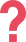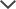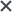The CogAT test pack provided my son with challenging questions, which prepared him to perform well on the test.
Subra
I got the Family Membership. It was excellent!
Velupillai
My kids were happy with this product and I would not change anything about it. The site is user-friendly and we had an overall great experience.
Ira
We used the OLSAT practice pack. We had a very good experience with this product. The study guide provided very useful information to familiarize with the test. I would give it a 9 out of 10.
Jingguo
I'm happy to report that my 3rd grade niece took her SCAT test and passed the quantitative math portion with flying colors - 44 of 50 correct! Your test preps were a huge help.
Anonymous

## 3rd Grade Math Practice Questions and Drills

In most elementary school math curricula, 3rd Grade is a crucial year. Students are expected to perform both long and short addition, subtraction, multiplication, and division. Number sense and geometric concepts introduced in grades K-2 are taught more formally, and presented as both number and word problems.

Help your third grader improve his or her understanding of 3rd grade math topics with our 3rd Grade Math Basic Pack. This pack is designed to enrich your child’s knowledge whilst covering an array of word problem topics including calculating arithmetic, distances, work rate, and geometry. The pack can be used either for extra practice or enrichment. Solutions including thorough explanations are provided for all questions. Concepts are presented in study guides, which also provide tips for solving different kinds of math problems. Topics covered include:

• Coins & Money
• Computation
• Math Sequences
• Place Value
• Price Lists
• Equations
• Estimation & Measurement
• 4 Operations
• Geometry
• Numbers & Operations
• Sequences
• Various Problems
• Word Problems

TestPrep-Online provides 204 questions and four math study guides for 3rd grade, which may also be used as enrichment for CogAT Level 9. Questions are divided into drills by topic so you can develop a practice plan for your child to strengthen his/her weaknesses or provide enrichment in topics that may not have been covered fully in the classroom. Drills can be practiced or reviewed any time, anywhere. The materials provided in the Prep Pack can serve as a basis for real-world practice, especially for money and measurement skills that involve tangible objects.

## Flexible Uses of 3rd Grade Math Tests (Level 9 Math)

The drills and study guides provided in the 3rd Grade Math pack can be used for a variety of purposes, including:

• Reviewing and reinforcing concepts that were difficult for your child in the classroom
• Enriching classroom instruction with more difficult questions at a higher level
• Preparing for classroom, state, and gifted education tests
• Maintaining skills during school vacations or extended absences
• Supporting a homeschool curriculum

With a 12-month license, you'll be able to use the materials to enhance your child's education all year long, covering and reviewing various topics as necessary.

## 3rd Grade Math Sample Questions

1. What number is missing from the sequence?

420  530  640  750  _?__

A. 830

B. 840

C. 850

D. 860

The correct answer is (D).

In order to solve this problem, find the common difference between each pair of numbers next to each other in the sequence:The common difference between each number in this sequence is +110.
Therefore, the number missing from the sequence is:
750 + 110 = 700 + 100 + 50 + 10 = 860
Thus, the correct answer is (D).

2.3. Which number is greater than 397 by 483?

A. 860

B. 870

C. 872

D. 880

Solutions with Explanations

1. The correct answer is (D).

In order to solve this problem, find the common difference between each pair of numbers next to each other in the sequence:The common difference between each number in this sequence is +110.
Therefore, the number missing from the sequence is:
750 + 110 = 700 + 100 + 50 + 10 = 860
Thus, the correct answer is (D).

2.

To solve this problem, sum the value of the coins and bills that appear in the picture. From left to right, there is a one-cent coin (penny), another one-cent coin, a ten-cent coin (dime), a one-dollar bill, a five-dollar bill, and another one-dollar bill:

\$1 + \$5 + \$1 + 1¢ + 1¢ + 10¢ = \$7 + 12¢ = \$7 + \$0.12 = \$7.12.

Thus, the correct answer is (A).

3.

The correct answer is (D).

There are two ways to solve this problem. The first way is to use vertical addition:Another way is to separately add the hundreds, tens, and ones and then sum the results:
397 + 483 = 300 + 400 + 90 + 80 + 7 + 3= 700 + 170 + 10 = 880. Thus, the correct answer is (D).

## Get the 3rd Grade Math Pack

For an additional 200+ math questions and extra study guides, check out our 3rd Grade Math Premium Pack. The 3rd Grade Math Premium Pack includes all of the 3rd Grade Math Basic Pack plus an additional 5 sets of Word Problems, 4 sets of Working with Numbers exercises, 3 sets of Extra Practice drills, and 2 additional Study Guides (Equations and Geometry). Upgrade to give your child more opportunities to strengthen his or knowledge. If you're also looking prepare your child for standardized state and gifted and talented testing, or would like materials at a different level for another child, our Family Membership is a great deal that gives you access to all of our test prep materials. Join and start practicing today!

CogAT®, OLSAT®, and MAP® are not trademarks and or property of TestPrep-Online. The trademark holder is not affiliated with TestPrep-Online or this website.

Need HelpNeed Help